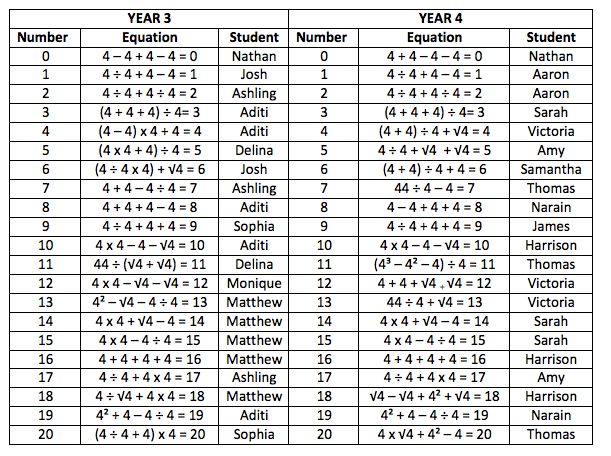#### You may also like### Pebbles

Place four pebbles on the sand in the form of a square. Keep adding as few pebbles as necessary to double the area. How many extra pebbles are added each time?### Bracelets

Investigate the different shaped bracelets you could make from 18 different spherical beads. How do they compare if you use 24 beads?### Sweets in a Box

How many different shaped boxes can you design for 36 sweets in one layer? Can you arrange the sweets so that no sweets of the same colour are next to each other in any direction?

# Four Goodness Sake

##### Age 7 to 11 Challenge Level:
Thank you for the large number of interesting solutions that came in. Of course your answers depended upon what you decided was 'allowed'.  For the first part, getting an answer of $12$, we had this variety of ideas:
$4^2 - 4 - 4 + 4$
$44+4\div4$
$(4-4/4)$$\times4$
$4+4+√4 + √4$
$4$ x $4 - √4 - √4$

From Year $3$ and $4$ Mathematics Extension Programme at Lumen Christi Catholic Primary School in Australia we had this very good solution:

To work out the solution to the problem we first tried using the four operations ($+, -, \times, \div$). This helped us find some of the solutions.
We then realised we would need to think about the order of operations to get different solutions. So we used our knowledge of brackets and BODMAS to find other solutions.
Finally we thought about other mathematical operators we could use to help us find more solutions.
We worked out that $4^2 = 16$; $4^3 = 64$ and $√4 = 2$ (square root $4$).
This information helped us find the remaining solutions except the answer.
Below are the solutions for the Year $3$ and Year $4$ Mathematics Extension Programs. Each group of students worked separately on the tasks, but have found some similar solutions.
Our goal is now to find all the solutions from $0 – 100$.Rosie Goodleigh C of E Primary School  sent in the following which was accompanied by similar solutions from Paige, George, Tom, Eva and Jessica.

$0$. First I added $4+4$ which made $8$. Then $-4$ which made $4$ and then$-4$ again which uses four $4$'s and makes $0$
$1$. First I did $4$ divided by $4$ which equals $1$ so then $+4$ and then $-4$ which makes $1$.
$2$. In brackets do $4$ divided by $4$, do this once more, both of them should
equal $1$ each,add both 1s together which equal $2$.
$3$. First do $4+4+4=12$. Subsequently divide $12$ by $4$ which equals $3$.
$4$. First take $4$ away from $4=0$. $0$x$4=0$,then add $4$ which equals $4$.
$5$. First times $4$ by $4 =16$ $16 + 4 =20$. Divide $20$ by $4=5$.
$6$.First add $4$ and $4 =8$. Divide $8$ by $4$ equals $2$. $2 + 4=6$.
$7$. In brackets divide $4$ by $4=1$. Then add $4$ and $4=8$ $- 1 =7$.
$8$. Add $4$ and $4$ x $4=32$. Divide $32$ by $4= 8$.
$9$. Divide $4$ by $4=1$. Add $4$ and $4=8$ $+ 1 = 9$.
$10$. In brackets there is the square root of $4$ which is $2$. Add $4$ and $4$ and $4 =12$ $-2=10$.
$12$. In brackets do $4$ divided by $4=1$. $4 - 1 = 3$. $3$x$4=12$.
$15$. In brackets do $4$ divided by $4 =1$. Do $4$ times $4$ then minus the $1$ to
equal $15$.
$16$. Add $4$ and $4$ and $4$ and $4 =16$.
$17$. In brackets divide $4$ by $4 =1$. Times $4$ by $4$ then add $1 =17$.

Sam from The Lantern Primary School sent in a really good alternative set of answers as follows.
The first ones I did I found quite easy. I used the four simple operations.
$1=(4 \div 4) \times (4 \div 4)$
$12=(44+4) \div 4$
$3=(4+4+4) \div 4$
$6=((4+4) \div 4)+4$
$7=(44 \div 4) −4$
$8=(4 \times 4) −4−4$
$9=(4 \div 4)+4+4$
$15=(4 \times 4) − (4 \div 4)$
$16 = 4+4+4+4$
$17 =(4 \times 4)+(4 \div 4)$

Then I came to a problem.  I couldn’t do 4, 5 and 10.  Then I realised that I had only used the simple operations, addition, subtraction, multiplication and division.  Then I remembered about square root, factorial and powers.  An example of factorial is:
$4!=4$×$3$×$2$×$1$

The first square root I tried was 4, then I realised I needed a few more.  Taking these into account, I was able to do the last few problems.
$4 = \sqrt(4+4+4+4)$
$5 = \sqrt(4!+(4 \div 4)^4)$
$10 = \sqrt(4!+(4 \div 4) \times 4)$

When I checked through my answers, I realised I had forgotten to put brackets in, so I added them in in the appropriate places to make the solutions clearer.

Thank you all for your excellent ideas which I think used so much of your mathematical knowledge and skills.